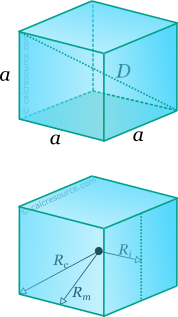## Properties of Cube

This tool calculates the basic geometric properties of a cube. Choose the known characteristic of the object and enter the appropriate value for it. The calculated results will have the same units as your input. Please use consistent units for any input.

 Known data: Edge length Inradius Circumradius Diagonal Midradius Geometric properties: Volume = Surface area = Face area = Edge length a = Diagonal D = Inradius Ri = Circumradius Rc = Midradius Rm = Number of faces = Number of edges = Number of vertices =## Definitions

### Geometry

The cube is a common 3-dimensional object (solid). It has 6 faces, 12 edges and 8 vertices. By definition, cube is a hexahedron (solid with six faces) and indeed is the unique regular hexahedron, where by regular is meant that all faces are identical regular polygons (squares for the cube).

The volume of a cube is given by the formula:

where a the length of its edges.

The total outer surface area of the cube can be easily found, considering that the area of one face is and there are six faces:

The interior diagonal of the cube (also called space diagonal), that passes through the cube centre, is equal to:

For any regular polyhedron, three spheres can be commonly defined: one that passes through all the vertices, called circumscribed sphere or circumsphere, one that passes through the centroids of all faces, called inscribed sphere or insphere, and one that passes through the middles of all edges, called midsphere. The radii of these spheres, circumradius , inradius and midradius , respectively, can be found for a cube, through the following expressions: